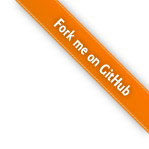# Application auto-scaling calculator

This tool helps you configuring your application for auto-scaling inside various orchestrators. It calculates the load of your application against a 'worst case scenario' and gives you back the key figures for your application's auto-scaling configuration.
You can find a more detailed description of the problematic and on how this tool works reading the article we published on the Toptal Engineering Blog: Do the Math: Scaling Microservices Applications with Orchestrators.

## Hypothesis

The instance start duration correspond to the time from when the scale-up is trigger to the time traffic gets routed to it.
The orchestrator will be considered stable at the begining of the load test (load has been constant).
Instance start duration:
in seconds
Minimum number of instances:

A load function translate the load of a single user over time (in seconds).
The time 't=0' correspond to the moment when the user starts rolling load test.
The function has to return a value in the same unit as the 'Instance maximal load' metric you filled above.
It should cover a time range from -Infinity to Infinity.
Pourcentage of user: %
JavaScript function:
in seconds

When using a Gaussian user distribution, the load test duration correspond to the duration during which 95.4% of users are performing the function.
Please refer to the article for a more detailed explication.
in seconds
Number of users:
User distribution function:

### Orchestrator

Following parameters are set on the kube-controller-manager service.
Beware that defaults values specified in the documentation are incorrects (but ones specified here are, you can check the source code).
Horizontal pod autoscaler sync period:
in seconds (defaults to 45 seconds)
Horizontal pod autoscaler tolerance:
between 0 and 1 (defaults to 0.1 (10%))
Horizontal pod autoscaler upscale delay:
in seconds (defaults to 1 minutes)
Horizontal pod autoscaler downscale delay:
in seconds (defaults to 2 minutes)
Following parameters are set on the kube-controller-manager service.
Horizontal pod autoscaler sync period:
in seconds (defaults to 15 seconds)
Horizontal pod autoscaler tolerance:
between 0 and 1 (defaults to 0.1 (10%))
in seconds (defaults to 30s)
Horizontal pod autoscaler downscale cooldown window:
in seconds (defaults to 5 minutes)
Interval:
in seconds
Autoscale multiplier:
Scale-up factor:
Scale-down factor:

### Computation

The number of iteration for the Riemann sum shouldn't be less than loadtest duration * number of points per second.
Number of points per second:
make sure this is (10 times) smaller than the smallest period of your load function
Number of iterations:
for the Riemann sum, the higher the more accurate (but the slower)## Results

%   (%)   (%)   (%, this is a maximum)

## How to interpret those results?

This value should be between 70 and 90 (the higher the better).

• If this value is below 70, here is a list of what you can do:
• increase the minimum number of instances
• increase resources to each instance
• lower application startup time
• work on your application performances
• If this value is above 90, it eather means that the load test scenario is not constrictive enough, or that you've just built the best application ever!

This value should be between 70 and 90 (the higher the better).

• If this value is below 70, here is a list of what you can do:
• increase the minimum number of instances
• increase resources to each instance
• lower application startup time
• work on your application performances
• If this value is above 90, it eather means that the load test scenario is not constrictive enough, or that you've just built the best application ever!

This value should be between 70 and 90 (the higher the better).

• If this value is below 70, here is a list of what you can do:
• increase the minimum number of instances
• increase resources to each instance
• decrease the scale up factor
• lower application startup time
• increase the autoscale multiplier
• work on your application performances
• lower your synchronisation interval (this will increase load of the marathon-autoscaler)
• If this value is above 90, it eather means that the load test scenario is not constrictive enough, or that you've just built the best application ever!

This value should be between 35 and 55 (the higher the better).

• If this value is below 35, here is a list of what you can do:
• increase the minimum number of instances
• decrease the autoscale multiplier
• find a way to increase AS_MAX_RANGE
• If this value is above 55, well done!

We remind you that these results do not take into account the time to spawn new VM instances (if relevant).
Nephely - Application auto-scaling calculator